Convective Heat Transfer: One Dimensional - 6

# Convective Heat Transfer: One Dimensional - 6 - Heat Transfer - Mechanical Engineering

3.4 Thermal insulation
We have seen how heat transfer is important in various situations. Previous discussion indicates that we are all the time interested in the flow of the heat from one point to another point. However, there are many systems; in fact it is a part of the system, in which we are interested to minimize the losses through heat transfer. For example, in a furnace we want to have high heat transfer inside the furnace; however we do not want any heat loss through the furnace wall. Thus to prevent the heat transfer from the furnace to the atmosphere a bad heat conductor or a very good heat insulator is required. In case of furnace the wall is prepared by multiple layers of refractory materials to minimize the heat losses. Therefore, wall insulation is required in various process equipment, reactors, pipelines etc. to minimize the heat loss from the system to the environment or heat gain from the environment to the system (like cryogenic systems). However, there are situations in which we want to maximize the losses for example, insulation to electric wires.

The petroleum conservation research association (PCRA) provides a good database on the properties and applications of industrial thermal insulations . The table 3.2 shows some common insulations used in chemical process industries for various process equipment and pipelines.

Table-3.2: Thermal properties of a few of the insulations being used in the chemical process industries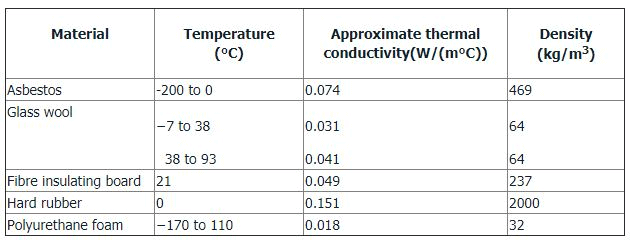An interesting application of the heat loss from a surface of some practical significance is found in the case of insulation of cylindrical surfaces like small pipes or electrical wires. In many a cases we desire to examine the variation in heat loss from the pipe with the change in insulation thickness, assuming that the length of the pipe is fixed. As insulation is added to the pipe, the outer exposed surface temperature will decrease, but at the same time the surface area available to the convective heat dissipation will increase. Therefore, it would be interesting to study these opposing effects.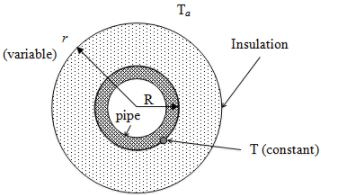Fig. 3.10: Heat dissipation from an insulated pipe

Let us consider a thick insulation layer which is installed around a cylindrical pipe as shown in fig. 3.10 (equivalent electrical circuit is shownin figure 3.11). Let the pipe radius be R and the insulation radius is r. This (r-R) will represent the thickness of the insulation. If the fluid carried by the pipe is at a temperature T and the ambient temperature is Ta. The insulation of the pipe will alter pipe surface temperature T in the radial direction. That is the temperature of the inner surface of the pipe and the outer surface (below insulation) of the pipe will be different. However, if the thermal resistance offered by the pipe is negligible, it can be considered that the temperature (T) is same across the pipe wall thickness and it is a common insulation case (please refer previous discussion). It can also be assumed that the heat transfer coefficient inside the pipe is very high as compared to the heat transfer coefficient at the outside of the insulated pipe. Therefore, only two major resistances in series will be available (insulation layer and gas film of the ambient).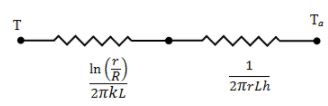Fig.3.11: Resistance offered by the insulation and ambient gas film

Therefore,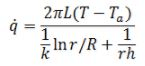where, k is the thermal conductivity of the material.
On differentiating above equation with respect to r will show that the heat dissipation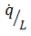reaches a maximum,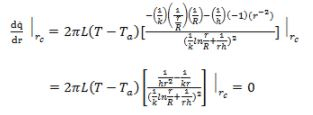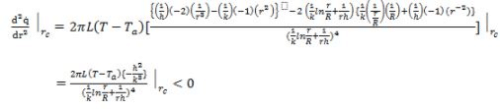So it is maxima, where the insulation radius is equal to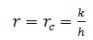where, rc denotes the critical radius of the insulation. The heat dissipation is maximum at rc which is the result of the previously mentioned opposing effects.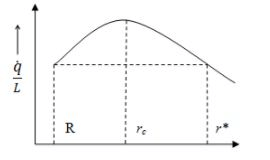Fig. 3.12: The critical insulation thickness of the pipe insulator

Therefore, the heat dissipation from a pipe increases by the addition of the insulation. However, above rc the heat dissipation reduces. The same is shown in fig. 3.12.

The careful analysis of the rc reveals that it is a fixed quantity determined by the thermal properties of the insulator. If R <r, then the initial addition of insulation will increase the heat loss until r =rcand after which it begins to decrease. The same heat dissipation which was at bare pipe radius is again attained at r*. The critical insulation thickness may not always exist for an insulated pipe, if the values of k and h are such that the ratio k/h turns out to be less than R.

It is clear from the above discussion that the insulation above rc reduces the heat dissipation from the cylindrical surface. However, if we keep on increasing the insulation the cost of insulation also increases. Thus again there are two opposing factors that must be considered to obtain the optimum thickness. It should be calculated that what is the pay-back period, that is in how many years the cost of insulation is recovered by the cost of energy saving.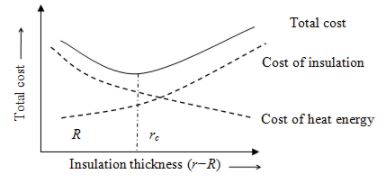Fig. 3.13: Optimum insulation thickness

The optimum insulation thickness (fig. 3.13) can be determined at which the sum of the insulation cost and the cost of the heat loss is minimum.

The document Convective Heat Transfer: One Dimensional - 6 | Heat Transfer - Mechanical Engineering is a part of the Mechanical Engineering Course Heat Transfer.
All you need of Mechanical Engineering at this link: Mechanical Engineering

## FAQs on Convective Heat Transfer: One Dimensional - 6 - Heat Transfer - Mechanical Engineering

 1. What is convective heat transfer?Ans. Convective heat transfer is the transfer of heat between a solid surface and a fluid (liquid or gas) in motion. It occurs due to the combined effects of conduction (heat transfer through a solid) and advection (heat transfer through fluid motion). In this process, the fluid carries heat away from the solid surface, resulting in temperature changes in both the fluid and the solid.
 2. What is one-dimensional heat transfer?Ans. One-dimensional heat transfer refers to the transfer of heat in a single direction, typically along a straight line. It assumes that heat transfer occurs only in one direction and that there are no variations in temperature or heat flux in the other two dimensions. This simplification is often used in engineering calculations to analyze heat transfer in systems with uniform cross-sectional areas, such as pipes or rods.
 3. How is convective heat transfer analyzed mathematically?Ans. Convective heat transfer can be mathematically analyzed using the concept of convective heat transfer coefficient, denoted as h. The convective heat transfer coefficient represents the rate at which heat is transferred per unit surface area and per unit temperature difference between the solid surface and the fluid. It can be determined experimentally or calculated using correlations based on fluid properties, flow conditions, and geometry.
 4. What factors affect convective heat transfer?Ans. Several factors influence convective heat transfer, including: - Fluid properties: The thermal conductivity, viscosity, and specific heat of the fluid affect its ability to transfer heat. - Flow velocity: Higher flow velocities result in increased convective heat transfer due to enhanced fluid mixing and increased contact between the fluid and the solid surface. - Surface roughness: Rough surfaces promote turbulence and enhance convective heat transfer compared to smooth surfaces. - Temperature difference: A larger temperature difference between the solid surface and the fluid leads to higher convective heat transfer rates. - Geometry and dimensions: The shape, size, and orientation of the solid surface impact convective heat transfer.
 5. What are some practical applications of convective heat transfer in chemical engineering?Ans. Convective heat transfer plays a crucial role in various chemical engineering processes, including: - Heat exchangers: Convective heat transfer is utilized in heat exchangers to efficiently transfer heat between two fluids, such as in refrigeration systems or industrial processes. - Cooling towers: Convective heat transfer is employed in cooling towers to remove excess heat from industrial processes or power plants. - Fluidized bed reactors: Convective heat transfer is essential in fluidized bed reactors, where solid particles are suspended and contacted with a fluid to carry out chemical reactions. - Distillation columns: Convective heat transfer is involved in the separation of mixtures in distillation columns, where heat is supplied or removed to achieve desired product purity. - Polymer processing: Convective heat transfer is crucial in polymer processing operations, such as extrusion or injection molding, to control the cooling and solidification of the polymer materials.

## Heat Transfer

58 videos|69 docs|86 tests

## Heat Transfer

58 videos|69 docs|86 tests
Signup to see your scores go up within 7 days! Learn & Practice with 1000+ FREE Notes, Videos & Tests.
10M+ students study on EduRev
Track your progress, build streaks, highlight & save important lessons and more!(Scan QR code)
Related Searches

,

,

,

,

,

,

,

,

,

,

,

,

,

,

,

,

,

,

,

,

,

;